# 11  Binary Logistic Regression Case Study 1

Case Study in Binary Logistic Regression, Model Selection and Approximation: Predicting Cause of Death

## 11.1 Overview

This chapter contains a case study on developing, describing, and validating a binary logistic regression model. In addition, the following methods are exemplified:

1. Data reduction using incomplete linear and nonlinear principal components
2. Use of AIC to choose from five modeling variations, deciding which is best for the number of parameters
3. Model simplification using stepwise variable selection and approximation of the full model
4. The relationship between the degree of approximation and the degree of predictive discrimination loss
5. Bootstrap validation that includes penalization for model uncertainty (variable selection) and that demonstrates a loss of predictive discrimination over the full model even when compensating for overfitting the full model.

The data reduction and pre-transformation methods used here were discussed in more detail in Chapter 8. Single imputation will be used because of the limited quantity of missing data.

## 11.2 Background

Consider the randomized trial of estrogen for treatment of prostate cancer described in Chapter 8. In this trial, larger doses of estrogen reduced the effect of prostate cancer but at the cost of increased risk of cardiovascular death. Kay (1986) did a formal analysis of the competing risks for cancer, cardiovascular, and other deaths. It can also be quite informative to study how treatment and baseline variables relate to the cause of death for those patients who died. We subset the original dataset of those patients dying from prostate cancer ($$n=130$$), heart or vascular disease ($$n=96$$), or cerebrovascular disease ($$n=31$$). Our goal is to predict cardiovascular–cerebrovascular death (cvd, $$n=127$$) given the patient died from either cvd or prostate cancer. Of interest is whether the time to death has an effect on the cause of death, and whether the importance of certain variables depends on the time of death.

## 11.3 Data Transformations and Single Imputation

In R, first obtain the desired subset of the data and do some preliminary calculations such as combining an infrequent category with the next category, and dichotomizing ekg for use in ordinary principal components (PCs).

Code
require(rms)
options(prType='html')
getHdata(prostate)
prostate <-
within(prostate, {
levels(ekg)[levels(ekg) %in%
c('old MI','recent MI')] <- 'MI'
ekg.norm <- 1*(ekg %in% c('normal','benign'))
levels(ekg) <- abbreviate(levels(ekg))
pfn <- as.numeric(pf)
levels(pf)  <- levels(pf)[c(1,2,3,3)]
cvd <- status %in% c("dead - heart or vascular",
rxn = as.numeric(rx) })
# Use transcan to compute optimal pre-transformations
ptrans <-   # See Figure (* @fig-prostate-transcan*)
transcan(~ sz + sg + ap + sbp + dbp +
age + wt + hg + ekg + pf + bm + hx + dtime + rx,
imputed=TRUE, transformed=TRUE,
data=prostate, pl=FALSE, pr=FALSE)
# Use transcan single imputations
imp <- impute(ptrans, data=prostate, list.out=TRUE)


Imputed missing values with the following frequencies
and stored them in variables with their original names:

sz  sg age  wt ekg
5  11   1   2   8 
Code
NAvars <- all.vars(~ sz + sg + age + wt + ekg)
for(x in NAvars) prostate[[x]] <- imp[[x]]
subset <- prostate$status %in% c("dead - heart or vascular", "dead - cerebrovascular","dead - prostatic ca") trans <- ptrans$transformed[subset,]
psub  <- prostate[subset,]

## 11.4 Regression on Original Variables, Principal Components and Pretransformations

We first examine the performance of data reduction in predicting the cause of death, similar to what we did for survival time in Section Section 8.6. The first analyses assess how well PCs (on raw and transformed variables) predict the cause of death.

There are 127 cvds. We use the 15:1 rule of thumb discussed in Section 4.4 to justify using the first 8 PCs. ap is log-transformed because of its extreme distribution. We use Hmisc::princmp.

Code
# Compute the first 8 PCs on raw variables then on
# transformed ones
p <- princmp(~ sz + sg + log(ap) + sbp + dbp + age +
wt + hg + ekg.norm + pfn + bm + hx + rxn + dtime,
data=psub, k=8, sw=TRUE, kapprox=2)
p
Principal Components Analysis

Stepwise Approximations to PCs With Cumulative R^2

PC 1
log(ap) (0.609) + bm (0.737) + dbp (0.803) + sg (0.86) + hg (0.901)

PC 2
dbp (0.371) + sg (0.544) + age (0.652) + sbp (0.743) + hx (0.803)
Code
plot(p)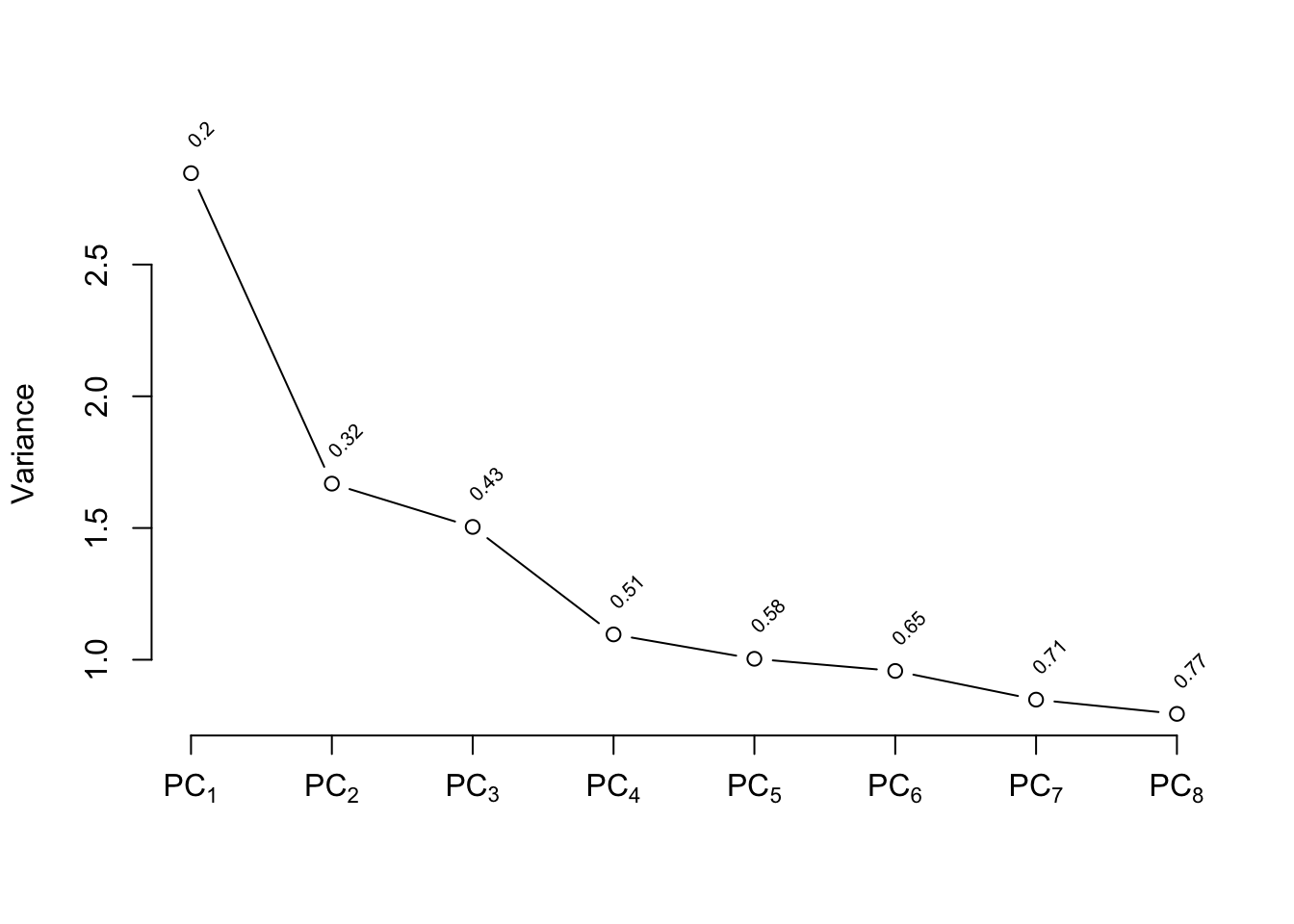Code
plot(p, 'loadings')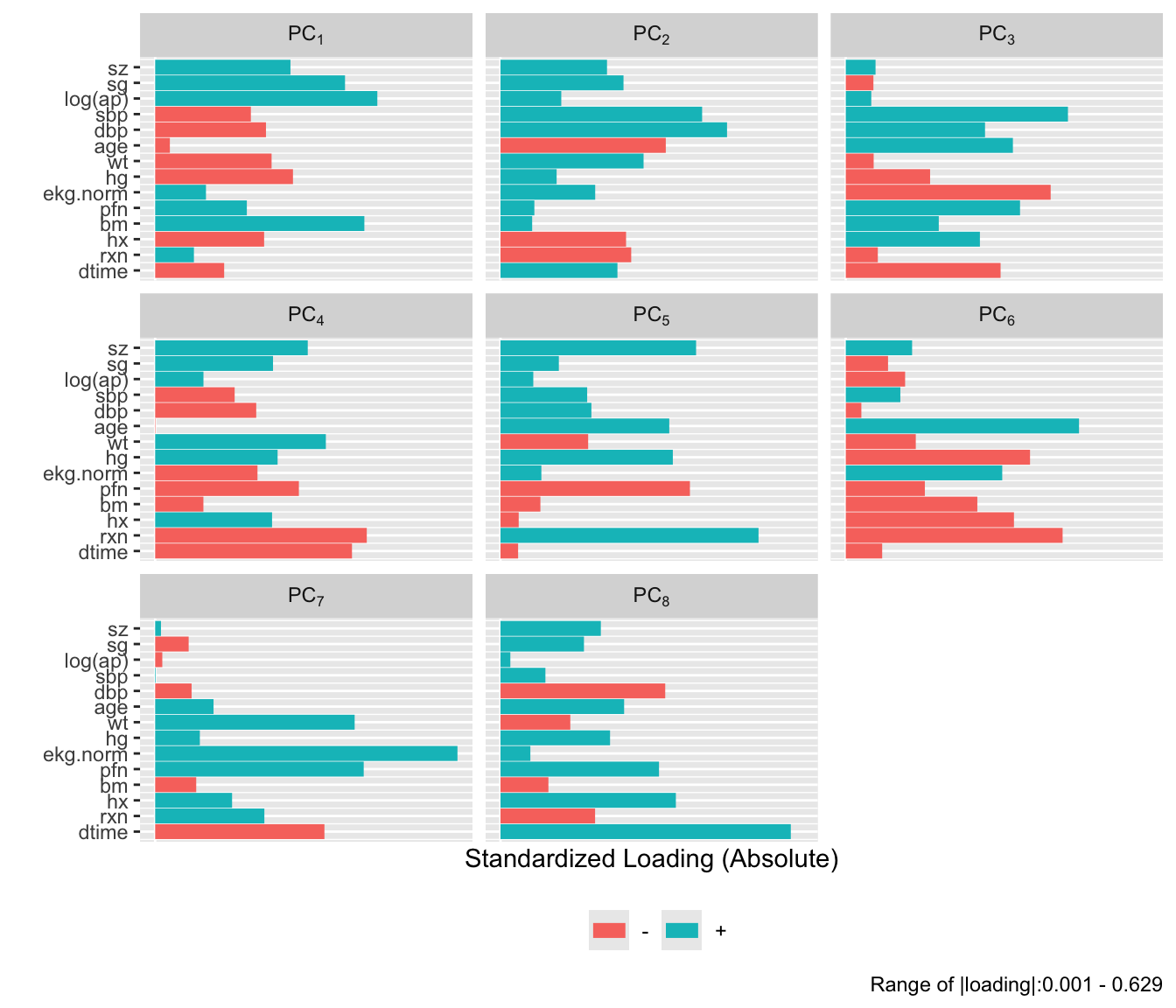Code
pc8 <- p$scores f8 <- lrm(cvd ~ pc8, data=psub) p <- princmp(trans, k=8, sw=TRUE, kapprox=2) p Principal Components Analysis Stepwise Approximations to PCs With Cumulative R^2 PC 1 ap (0.633) + bm (0.744) + dbp (0.811) + sg (0.863) + hg (0.904) PC 2 sbp (0.499) + pf (0.692) + ekg (0.806) + bm (0.861) + dbp (0.901) Code plot(p)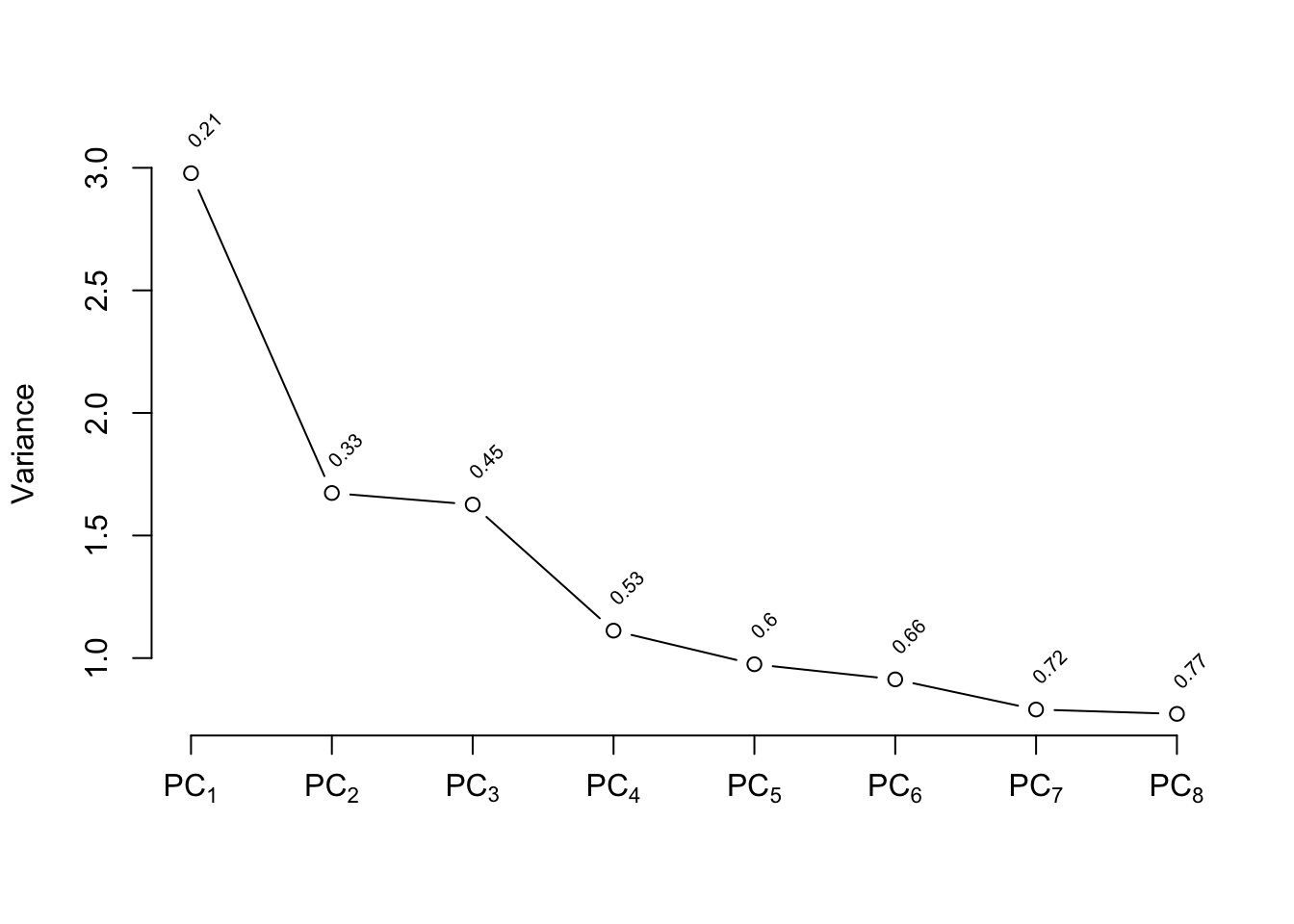Code plot(p, 'loadings')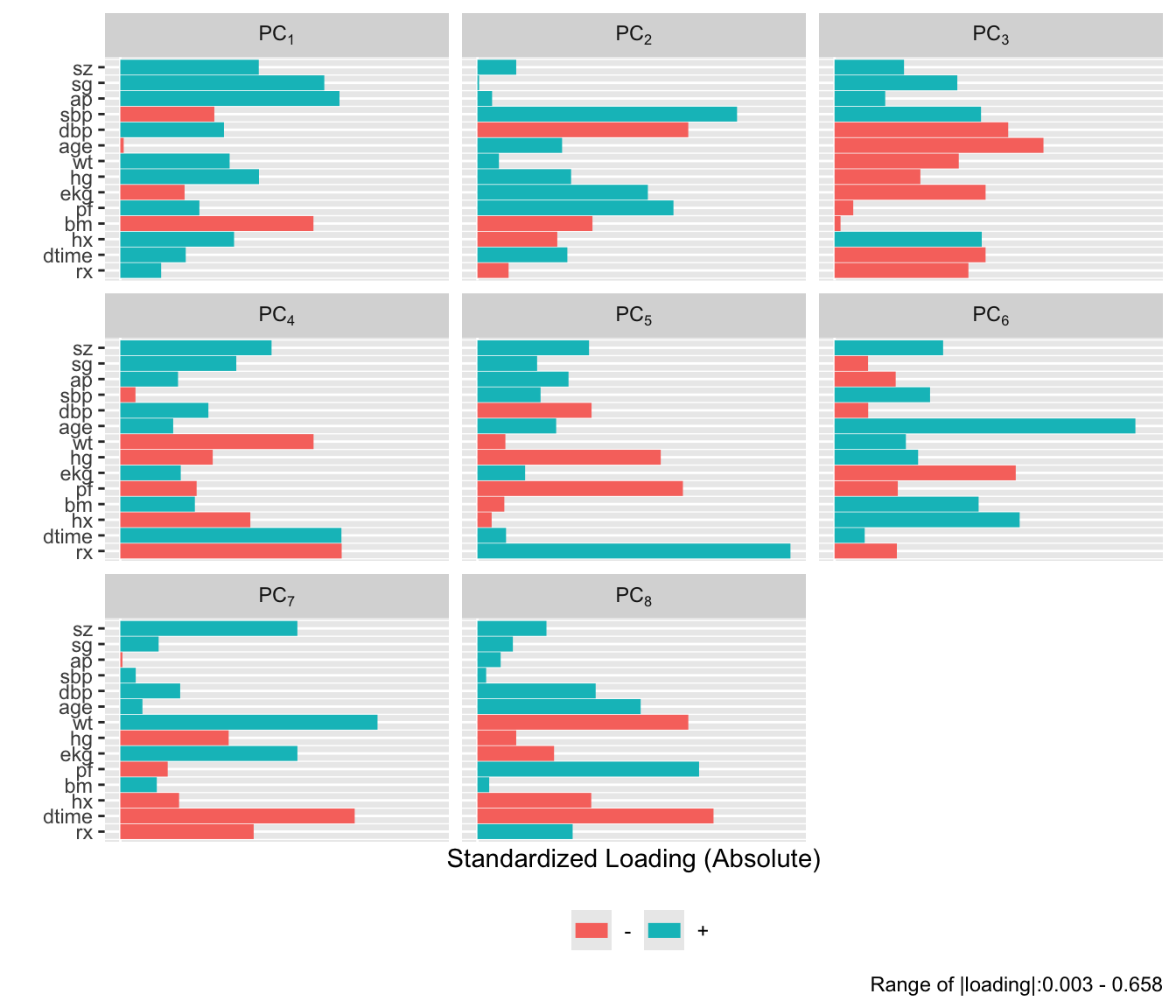Code pc8t <- p$scores
f8t  <- lrm(cvd ~ pc8t, data=psub)
# Fit binary logistic model on original variables
# x=TRUE y=TRUE are for test='LR', validate, calibrate
f <- lrm(cvd ~ sz + sg + log(ap) + sbp + dbp + age +
wt + hg + ekg + pf + bm + hx + rx + dtime,
x=TRUE, y=TRUE, data=psub)
# Expand continuous variables using splines
g <- lrm(cvd ~ rcs(sz,4) + rcs(sg,4) + rcs(log(ap),4) +
rcs(sbp,4) + rcs(dbp,4) + rcs(age,4) + rcs(wt,4) +
rcs(hg,4) + ekg + pf + bm + hx + rx + rcs(dtime,4),
data=psub)
# Fit binary logistic model on individual transformed var.
h <- lrm(cvd ~ trans, data=psub)

The five approaches to modeling the outcome are compared using AIC (where smaller is better).

Code
c(f8=AIC(f8), f8t=AIC(f8t), f=AIC(f), g=AIC(g), h=AIC(h))
      f8      f8t        f        g        h
257.6573 254.5172 255.8545 263.8413 254.5317 

Based on AIC, the more traditional model fitted to the raw data and assuming linearity for all the continuous predictors has only a slight chance of producing worse cross-validated predictive accuracy than other methods. The chances are also good that effect estimates from this simple model will have competitive mean squared errors.

## 11.5 Description of Fitted Model

Here we describe the simple all-linear full model. Summary statistics and Wald and likelihood ratio ANOVA tables are below, followed by partial effects plots with pointwise confidence bands, and odds ratios over default ranges of predictors.

Code
f

Logistic Regression Model

lrm(formula = cvd ~ sz + sg + log(ap) + sbp + dbp + age + wt +
hg + ekg + pf + bm + hx + rx + dtime, data = psub, x = TRUE,
y = TRUE)

Model Likelihood
Ratio Test
Discrimination
Indexes
Rank Discrim.
Indexes
Obs 257 LR χ2 144.39 R2 0.573 C 0.893
FALSE 130 d.f. 21 R221,257 0.381 Dxy 0.786
TRUE 127 Pr(>χ2) <0.0001 R221,192.7 0.473 γ 0.786
max |∂log L/∂β| 5×10-11 Brier 0.133 τa 0.395
β S.E. Wald Z Pr(>|Z|)
Intercept  -4.5130  3.2210 -1.40 0.1612
sz  -0.0640  0.0168 -3.80 0.0001
sg  -0.2967  0.1149 -2.58 0.0098
ap  -0.3927  0.1411 -2.78 0.0054
sbp  -0.0572  0.0890 -0.64 0.5201
dbp   0.3917  0.1629 2.40 0.0162
age   0.0926  0.0286 3.23 0.0012
wt  -0.0177  0.0140 -1.26 0.2069
hg   0.0860  0.0925 0.93 0.3524
ekg=bngn   1.0781  0.8793 1.23 0.2202
ekg=rd&ec  -0.1929  0.6318 -0.31 0.7601
ekg=hbocd  -1.3679  0.8279 -1.65 0.0985
ekg=hrts   0.4365  0.4582 0.95 0.3407
ekg=MI   0.3039  0.5618 0.54 0.5886
pf=in bed < 50% daytime   0.9604  0.6956 1.38 0.1673
pf=in bed > 50% daytime  -2.3232  1.2464 -1.86 0.0623
bm   0.1456  0.5067 0.29 0.7738
hx   1.0913  0.3782 2.89 0.0039
rx=0.2 mg estrogen  -0.3022  0.4908 -0.62 0.5381
rx=1.0 mg estrogen   0.7526  0.5272 1.43 0.1534
rx=5.0 mg estrogen   0.6868  0.5043 1.36 0.1733
dtime  -0.0136  0.0107 -1.27 0.2040
Code
anova(f)
Wald Statistics for cvd
χ2 d.f. P
sz 14.42 1 0.0001
sg 6.67 1 0.0098
ap 7.74 1 0.0054
sbp 0.41 1 0.5201
dbp 5.78 1 0.0162
age 10.45 1 0.0012
wt 1.59 1 0.2069
hg 0.86 1 0.3524
ekg 6.76 5 0.2391
pf 5.52 2 0.0632
bm 0.08 1 0.7738
hx 8.33 1 0.0039
rx 5.72 3 0.1260
dtime 1.61 1 0.2040
TOTAL 66.87 21 <0.0001
Code
an <- anova(f, test='LR')
an
Likelihood Ratio Statistics for cvd
χ2 d.f. P
sz 17.81 1 <0.0001
sg 6.96 1 0.0083
ap 8.29 1 0.0040
sbp 0.42 1 0.5190
dbp 6.00 1 0.0143
age 11.52 1 0.0007
wt 1.60 1 0.2056
hg 0.85 1 0.3554
ekg 7.08 5 0.2146
pf 7.12 2 0.0285
bm 0.08 1 0.7736
hx 8.58 1 0.0034
rx 5.97 3 0.1133
dtime 1.64 1 0.2003
TOTAL 144.39 21 <0.0001
Code
spar(ps=8,top=0.5)
plot(an)
s <- f$stats gamma.hat <- (s['Model L.R.'] - s['d.f.'])/s['Model L.R.']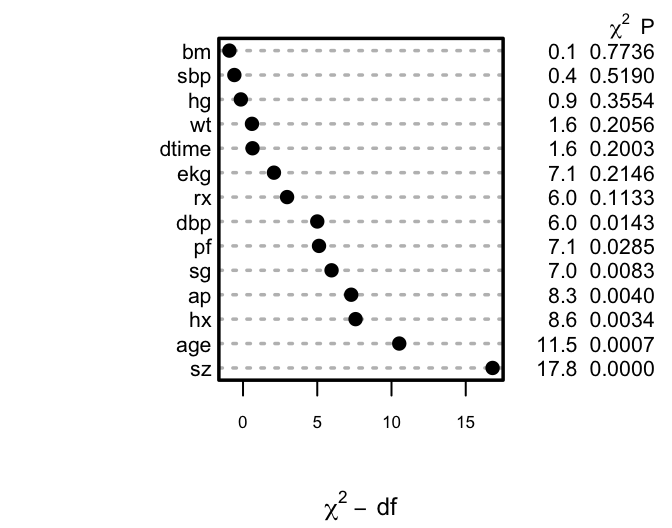Figure 11.1: Ranking of apparent importance of predictors of cause of death using LR statistics Code dd <- datadist(psub); options(datadist='dd') ggplot(Predict(f), sepdiscrete='vertical', vnames='names', rdata=psub, histSpike.opts=list(frac=function(f) .1*f/max(f) ))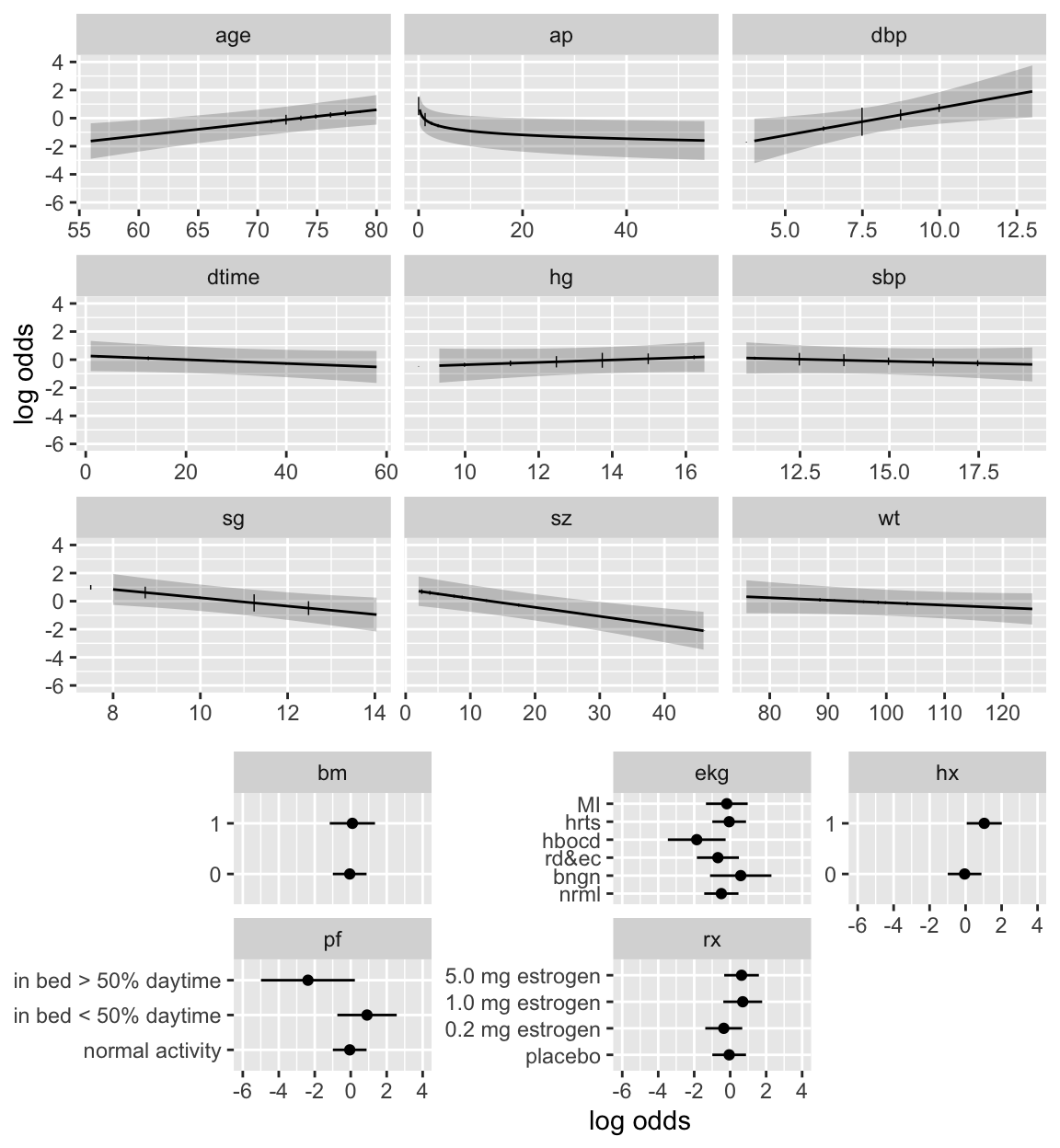Figure 11.2: Partial effects (log odds scale) in full model for cause of death, along with vertical line segments showing the raw data distribution of predictors Code plot(summary(f), log=TRUE)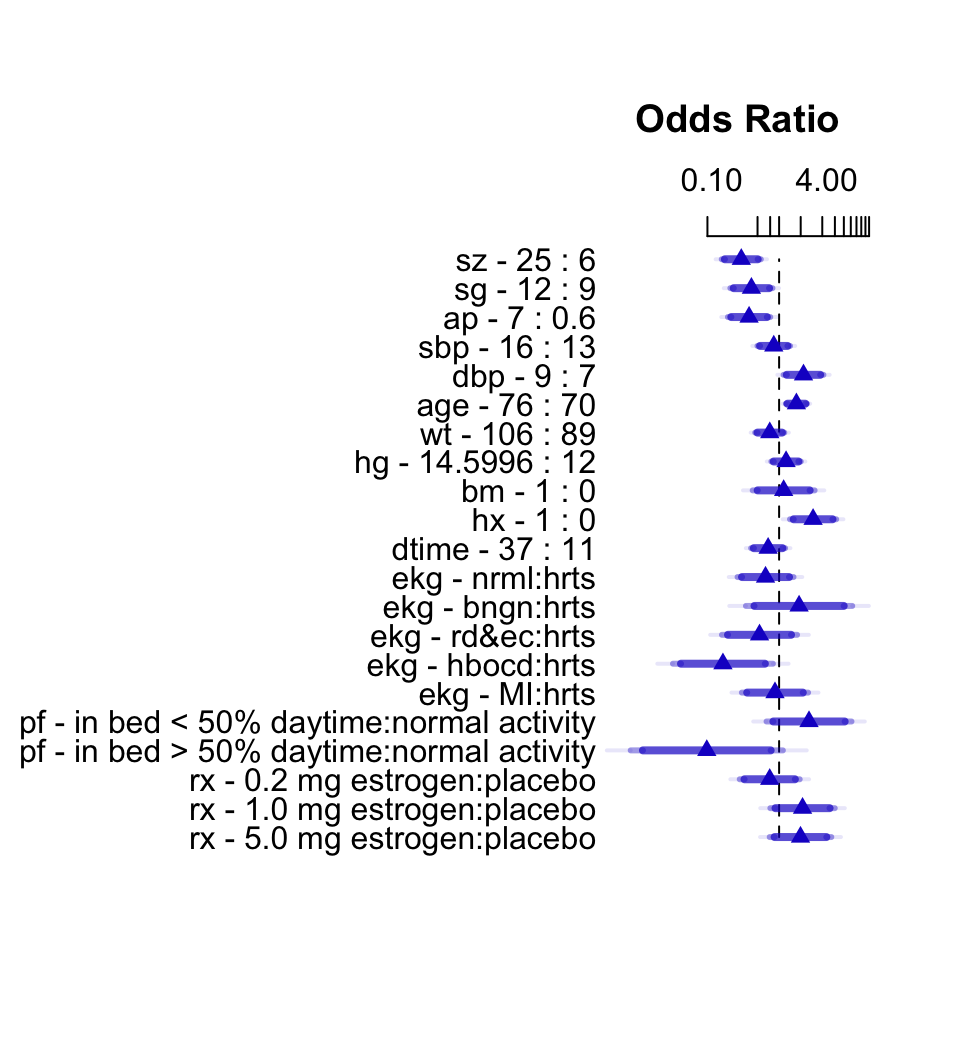Figure 11.3: Interquartile-range odds ratios for continuous predictors and simple odds ratios for categorical predictors. Numbers at left are upper quartile : lower quartile or current group : reference group. The bars represent $$0.9, 0.95, 0.99$$ confidence limits. The intervals are drawn on the log odds ratio scale and labeled on the odds ratio scale. Ranges are on the original scale. The van Houwelingen–Le Cessie heuristic shrinkage estimate (Equation 4.1) is $$\hat{\gamma}=0.85$$, indicating that this model will validate on new data about 15% worse than on this dataset. ## 11.6 Backwards Step-Down Now use fast backward step-down (with total residual AIC as the stopping rule) to identify the variables that explain the bulk of the cause of death. Later validation will take this screening of variables into account. The greatly reduced model results in a simple nomogram. Code fastbw(f)  Deleted Chi-Sq d.f. P Residual d.f. P AIC ekg 6.76 5 0.2391 6.76 5 0.2391 -3.24 bm 0.09 1 0.7639 6.85 6 0.3349 -5.15 hg 0.38 1 0.5378 7.23 7 0.4053 -6.77 sbp 0.48 1 0.4881 7.71 8 0.4622 -8.29 wt 1.11 1 0.2932 8.82 9 0.4544 -9.18 dtime 1.47 1 0.2253 10.29 10 0.4158 -9.71 rx 5.65 3 0.1302 15.93 13 0.2528 -10.07 pf 4.78 2 0.0915 20.71 15 0.1462 -9.29 sg 4.28 1 0.0385 25.00 16 0.0698 -7.00 dbp 5.84 1 0.0157 30.83 17 0.0209 -3.17 Approximate Estimates after Deleting Factors Coef S.E. Wald Z P Intercept -3.74986 1.82887 -2.050 0.0403286 sz -0.04862 0.01532 -3.174 0.0015013 ap -0.40694 0.11117 -3.660 0.0002518 age 0.06000 0.02562 2.342 0.0191701 hx 0.86969 0.34339 2.533 0.0113198 Factors in Final Model  sz ap age hx  Code fred <- lrm(cvd ~ sz + log(ap) + age + hx, data=psub) latex(fred) $\Pr(\mathrm{cvd}) = \frac{1}{1+\exp(-X\beta)}, \mathrm{~~where} \\$ $\begin{array} \lefteqn{X\hat{\beta}=}\\ & & -5.009276 -0.05510121\:\mathrm{sz} -0.509185\:\mathrm{\log(ap)} +0.0788052\:\mathrm{age} +1.070601\:\mathrm{hx} \\ \end{array}$ Code nom <- nomogram(fred, ap=c(.1, .5, 1, 5, 10, 50), fun=plogis, funlabel="Probability", fun.at=c(.01,.05,.1,.25,.5,.75,.9,.95,.99)) plot(nom, xfrac=.45)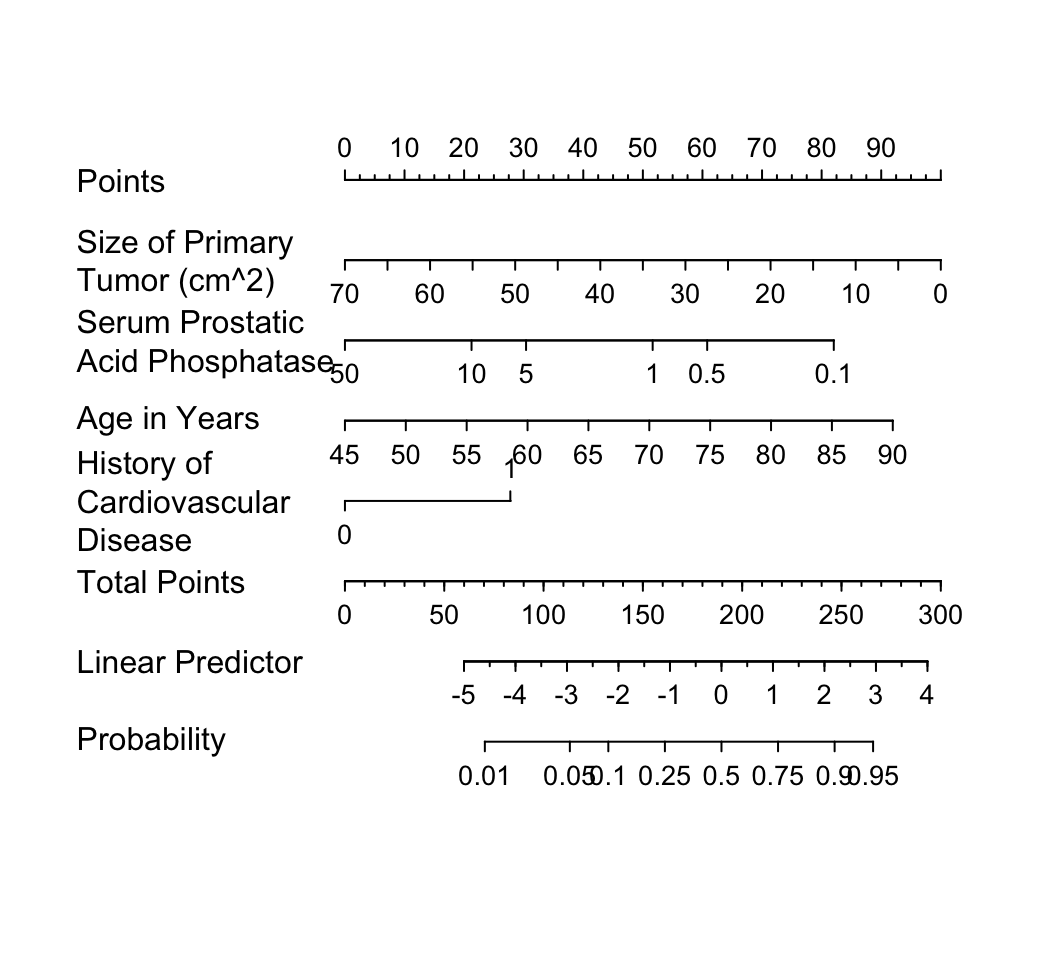Figure 11.4: Nomogram calculating $$X\hat{\beta}$$ and $$\hat{P}$$ for cvd as the cause of death, using the step-down model. For each predictor, read the points assigned on the 0–100 scale and add these points. Read the result on the Total Points scale and then read the corresponding predictions below it. It is readily seen from this model that patients with a history of heart disease, and patients with less extensive prostate cancer are those more likely to die from cvd rather than from cancer. But beware that it is easy to over-interpret findings when using unpenalized estimation, and confidence intervals are too narrow. Let us use the bootstrap to study the uncertainty in the selection of variables and to penalize for this uncertainty when estimating predictive performance of the model. The variables selected in the first 20 bootstrap resamples are shown, making it obvious that the set of “significant” variables, i.e., the final model, is somewhat arbitrary. Code v <- validate(f, B=200, bw=TRUE) Code print(v, B=20, digits=3) Index Original Sample Training Sample Test Sample Optimism Corrected Index Successful Resamples Dxy 0.682 0.714 0.647 0.068 0.614 200 R2 0.439 0.482 0.396 0.087 0.352 200 Intercept 0 0 0.002 -0.002 0.002 200 Slope 1 1 0.816 0.184 0.816 200 Emax 0 0 0.046 0.046 0.046 200 D 0.395 0.449 0.349 0.1 0.295 200 U -0.008 -0.008 0.015 -0.022 0.015 200 Q 0.403 0.457 0.335 0.122 0.281 200 B 0.162 0.151 0.172 -0.021 0.183 200 g 1.932 2.205 1.765 0.44 1.492 200 gp 0.341 0.356 0.322 0.034 0.307 200 Factors Retained in Backwards Elimination First 20 Resamples sz sg ap sbp dbp age wt hg ekg pf bm hx rx dtime Frequencies of Numbers of Factors Retained 1 2 3 4 5 6 7 8 9 11 12 2 44 51 44 26 13 10 4 4 1 1 The slope shrinkage ($$\hat{\gamma}$$) is a bit lower than was estimated above. There is drop-off in all indexes. The estimated likely future predictive discrimination of the model as measured by Somers’ $$D_{xy}$$ fell from 0.682 to 0.614. The latter estimate is the one that should be claimed when describing model performance. A nearly unbiased estimate of future calibration of the stepwise-derived model is given below. Code spar(ps=9, bot=1) cal <- calibrate(f, B=200, bw=TRUE)  Backwards Step-down - Original Model Deleted Chi-Sq d.f. P Residual d.f. P AIC ekg 6.76 5 0.2391 6.76 5 0.2391 -3.24 bm 0.09 1 0.7639 6.85 6 0.3349 -5.15 hg 0.38 1 0.5378 7.23 7 0.4053 -6.77 sbp 0.48 1 0.4881 7.71 8 0.4622 -8.29 wt 1.11 1 0.2932 8.82 9 0.4544 -9.18 dtime 1.47 1 0.2253 10.29 10 0.4158 -9.71 rx 5.65 3 0.1302 15.93 13 0.2528 -10.07 pf 4.78 2 0.0915 20.71 15 0.1462 -9.29 sg 4.28 1 0.0385 25.00 16 0.0698 -7.00 dbp 5.84 1 0.0157 30.83 17 0.0209 -3.17 Approximate Estimates after Deleting Factors Coef S.E. Wald Z P Intercept -3.74986 1.82887 -2.050 0.0403286 sz -0.04862 0.01532 -3.174 0.0015013 ap -0.40694 0.11117 -3.660 0.0002518 age 0.06000 0.02562 2.342 0.0191701 hx 0.86969 0.34339 2.533 0.0113198 Factors in Final Model  sz ap age hx  Code plot(cal)  n=257 Mean absolute error=0.03 Mean squared error=0.00112 0.9 Quantile of absolute error=0.054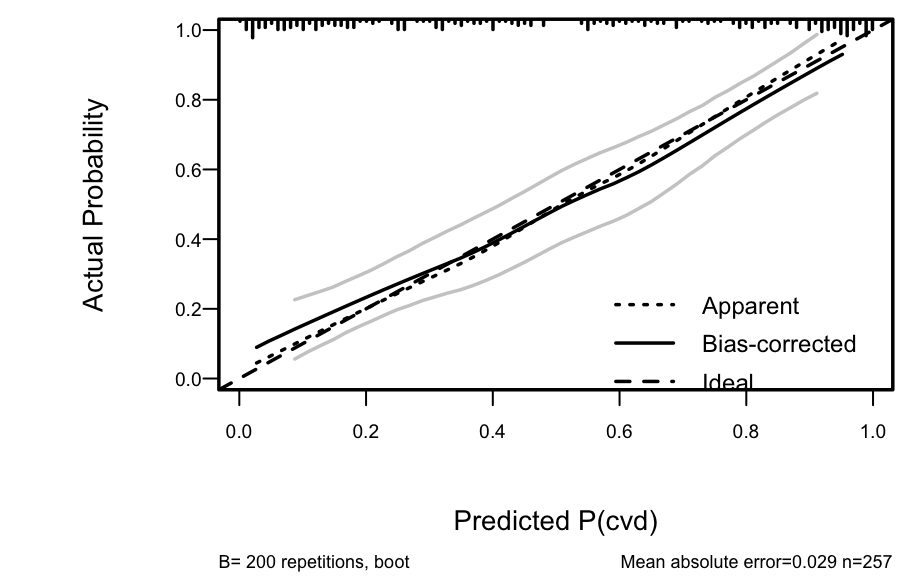Figure 11.5: Bootstrap overfitting-corrected calibration curve estimate for the backwards step-down cause of death logistic model, along with a rug plot showing the distribution of predicted risks. The smooth nonparametric calibration estimator (loess) is used. The amount of overfitting seen in Figure 11.5 is consistent with the indexes produced by the validate function. For comparison, consider a bootstrap validation of the full model without using variable selection. Code vfull <- validate(f, B=200) print(vfull, digits=3) Index Original Sample Training Sample Test Sample Optimism Corrected Index Successful Resamples Dxy 0.786 0.827 0.741 0.086 0.7 200 R2 0.573 0.633 0.504 0.129 0.445 200 Intercept 0 0 0.004 -0.004 0.004 200 Slope 1 1 0.707 0.293 0.707 200 Emax 0 0 0.078 0.078 0.078 200 D 0.558 0.641 0.471 0.169 0.389 200 U -0.008 -0.008 0.044 -0.052 0.044 200 Q 0.566 0.649 0.427 0.221 0.344 200 B 0.133 0.117 0.149 -0.032 0.165 200 g 2.688 3.404 2.387 1.018 1.67 200 gp 0.394 0.413 0.367 0.046 0.348 200 Compared to the validation of the full model, the step-down model has less optimism, but it started with a smaller $$D_{xy}$$ due to loss of information from removing moderately important variables. The improvement in optimism was not enough to offset the effect of eliminating variables. If shrinkage were used with the full model, it would have better calibration and discrimination than the reduced model, since shrinkage does not diminish $$D_{xy}$$. Thus stepwise variable selection failed at delivering excellent predictive discrimination. Finally, compare previous results with a bootstrap validation of a step-down model using a better significance level for a variable to stay in the model ($$\alpha=0.5$$, Steyerberg et al. (2000)) and using individual approximate Wald tests rather than tests combining all deleted variables. Code v5 <- validate(f, bw=TRUE, sls=0.5, type='individual', B=200)  Backwards Step-down - Original Model Deleted Chi-Sq d.f. P Residual d.f. P AIC ekg 6.76 5 0.2391 6.76 5 0.2391 -3.24 bm 0.09 1 0.7639 6.85 6 0.3349 -5.15 hg 0.38 1 0.5378 7.23 7 0.4053 -6.77 sbp 0.48 1 0.4881 7.71 8 0.4622 -8.29 wt 1.11 1 0.2932 8.82 9 0.4544 -9.18 dtime 1.47 1 0.2253 10.29 10 0.4158 -9.71 rx 5.65 3 0.1302 15.93 13 0.2528 -10.07 Approximate Estimates after Deleting Factors Coef S.E. Wald Z P Intercept -4.86308 2.67292 -1.819 0.068852 sz -0.05063 0.01581 -3.202 0.001366 sg -0.28038 0.11014 -2.546 0.010903 ap -0.24838 0.12369 -2.008 0.044629 dbp 0.28288 0.13036 2.170 0.030008 age 0.08502 0.02690 3.161 0.001572 pf=in bed < 50% daytime 0.81151 0.66376 1.223 0.221485 pf=in bed > 50% daytime -2.19885 1.21212 -1.814 0.069670 hx 0.87834 0.35203 2.495 0.012592 Factors in Final Model  sz sg ap dbp age pf hx  Code print(v5, digits=3, B=0) Index Original Sample Training Sample Test Sample Optimism Corrected Index Successful Resamples Dxy 0.739 0.802 0.717 0.084 0.655 200 R2 0.517 0.597 0.481 0.116 0.401 200 Intercept 0 0 0.003 -0.003 0.003 200 Slope 1 1 0.745 0.255 0.745 200 Emax 0 0 0.066 0.066 0.066 200 D 0.486 0.593 0.445 0.148 0.338 200 U -0.008 -0.008 0.032 -0.04 0.032 200 Q 0.494 0.6 0.412 0.188 0.306 200 B 0.147 0.125 0.155 -0.03 0.177 200 g 2.351 2.982 2.187 0.795 1.556 200 gp 0.372 0.4 0.358 0.042 0.33 200 The performance statistics are midway between the full model and the smaller stepwise model. ## 11.7 Model Approximation Frequently a better approach than stepwise variable selection is to approximate the full model, using its estimates of precision, as discussed in Section 5.5. Stepwise variable selection as well as regression trees are useful for making the approximations, and the sacrifice in predictive accuracy is always apparent. We begin by computing the “gold standard” linear predictor from the full model fit ($$R^{2} = 1.0$$), then running backwards step-down OLS regression to approximate it. Code spar(bty='l', ps=9) lp <- predict(f) # Compute linear predictor from full model # Insert sigma=1 as otherwise sigma=0 will cause problems a <- ols(lp ~ sz + sg + log(ap) + sbp + dbp + age + wt + hg + ekg + pf + bm + hx + rx + dtime, sigma=1, data=psub) # Specify silly stopping criterion to remove all variables s <- fastbw(a, aics=10000) betas <- s$Coefficients   # matrix, rows=iterations
X     <- cbind(1, f$x) # design matrix # Compute the series of approximations to lp ap <- X %*% t(betas) # For each approx. compute approximation R^2 and ratio of # likelihood ratio chi-square for approximate model to that # of original model m <- ncol(ap) - 1 # all but intercept-only model r2 <- frac <- numeric(m) fullchisq <- f$stats['Model L.R.']
for(i in 1:m) {
lpa <- ap[,i]
r2[i] <- cor(lpa, lp)^2
fapprox <- lrm(cvd ~ lpa, data=psub)
frac[i] <- fapprox$stats['Model L.R.'] / fullchisq } plot(r2, frac, type='b', xlab=expression(paste('Approximation ', R^2)), ylab=expression(paste('Fraction of ', chi^2, ' Preserved'))) abline(h=.95, col=gray(.83)); abline(v=.95, col=gray(.83)) abline(a=0, b=1, col=gray(.83))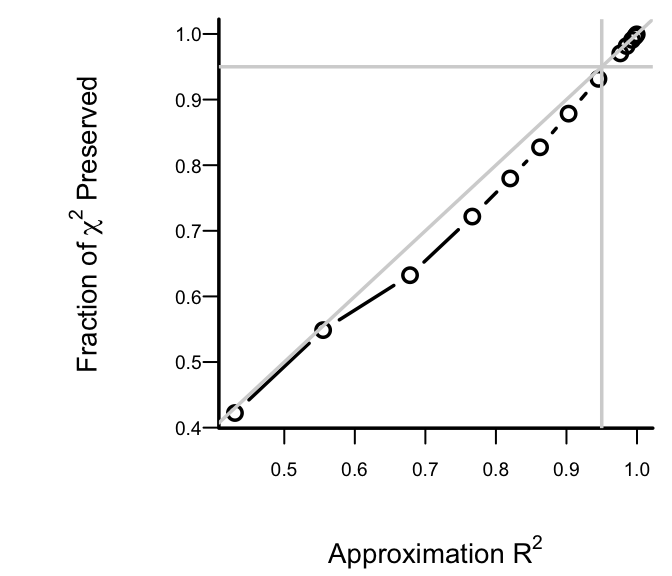Figure 11.6: Fraction of explainable variation (full model LR $$\chi^2$$) in cvd that was explained by approximate models, along with approximation accuracy ($$x$$-axis) After 6 deletions, slightly more than 0.05 of both the LR $$\chi^2$$ and the approximation $$R^2$$ are lost. Therefore we take as our approximate model the one that removed 6 predictors. The equation for this model is below, and its nomogram is in the figure below. Code fapprox <- ols(lp ~ sz + sg + log(ap) + age + ekg + pf + hx + rx, data=psub) fapprox$stats['R2']   # as a check
       R2
0.9453396 
Code
latex(fapprox)
$\mathrm{E}(\mathrm{lp}) = X\beta,~~\mathrm{where}$ $\begin{array} \lefteqn{X\hat{\beta}=}\\ & & -2.868303 -0.06233241\:\mathrm{sz} -0.3157901\:\mathrm{sg} -0.3834479\:\mathrm{\log(ap)} +0.09089393\:\mathrm{age} \\ & & +1.396922 [\mathrm{bngn}]+0.06275034[\mathrm{rd\&ec}]-1.24892 [\mathrm{hbocd}]+0.6511938 [\mathrm{hrts}]+0.3236771 [\mathrm{MI}] \\ & & +1.116028 [\mathrm{in\ bed\ <\ 50\%\ daytime}]-2.436734[\mathrm{in\ bed\ >\ 50\%\ daytime}] \\ & & +1.05316\:\mathrm{hx} \\ & & -0.3888534[\mathrm{0.2\ mg\ estrogen}]+0.6920495 [\mathrm{1.0\ mg\ estrogen}] \\ & & +0.7834498 [\mathrm{5.0\ mg\ estrogen}] \\ \end{array}$

$[c]=1~\mathrm{if~subject~is~in~group}~c,~0~\mathrm{otherwise}$

Code
spar(ps=8)
nom <- nomogram(fapprox, ap=c(.1, .5, 1, 5, 10, 20, 30, 40),
fun=plogis, funlabel="Probability",
lp.at=(-5):4,
fun.lp.at=qlogis(c(.01,.05,.25,.5,.75,.95,.99)))
plot(nom, xfrac=.45)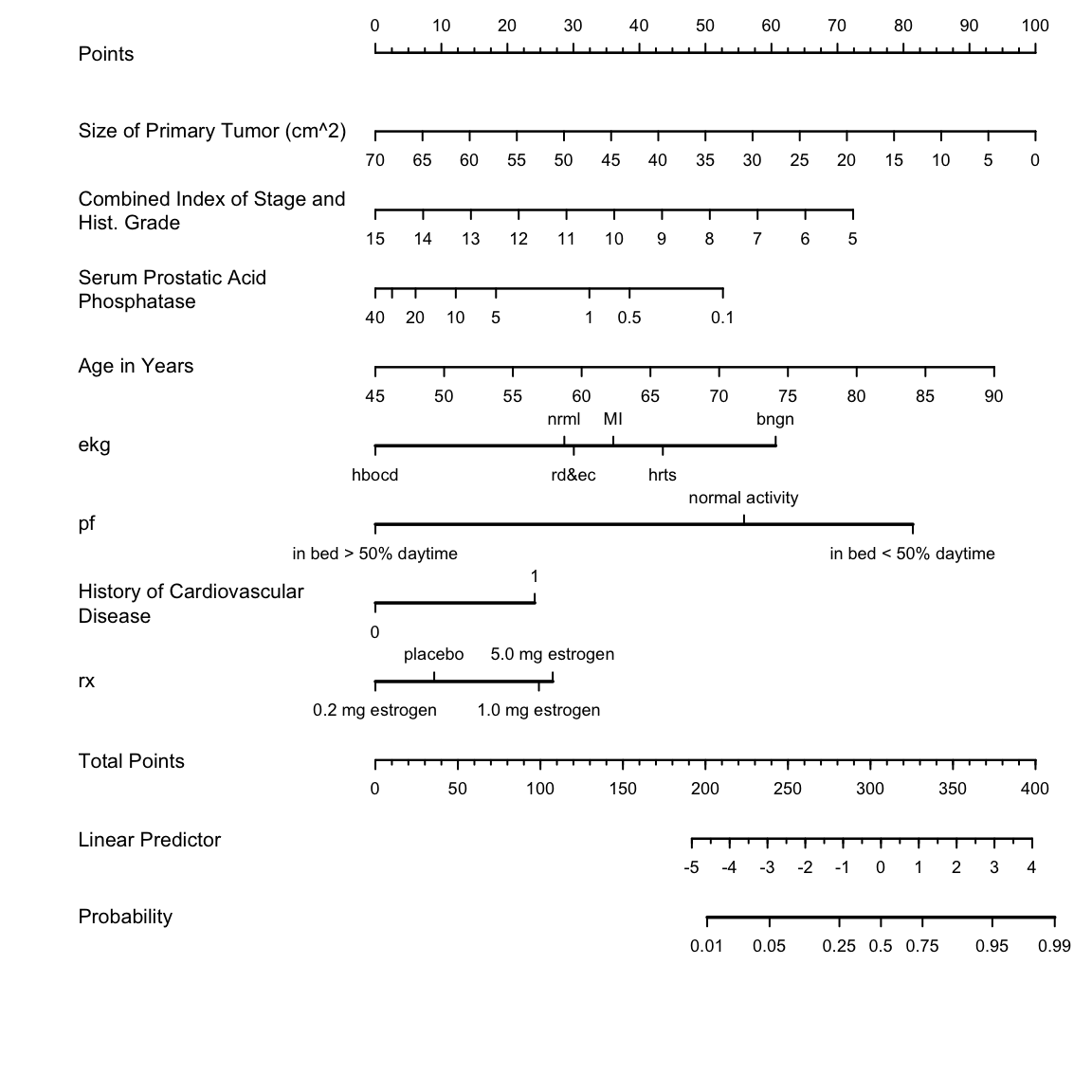Figure 11.7: Nomogram for predicting the probability of cvd based on the approximate model